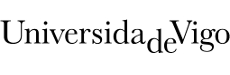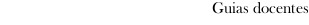Guia docente 2019_20Escuela de Ingeniería Industrial
 Degree in Mechanical EngineeringSubjectsResistance of materialsContents
 Topic Sub-topic 1. Introduction 1.1 Introduction 1.2 Review of statics fundamentals and applied concepts for further progress in solid mechanics and stress analysis 2. Basic principles of elasticity and mechanics of materials. 2.0 Stress and strain. Linear elastic materials 2.1. Normal stress in an axially loaded prismatic bar. 2.2. Equilibrium of a deformable body. 2.3. Stress-Strain diagram of ductile materials. Hookes Law. 2.4. Stress resultants. Diagrams. 3. Axial loads 3.1. Normal forces. 3.2. Elastic deformation of an axially loaded member. 3.3. Statically governed problems. 3.4. Statically indeterminate problems. 3.5. Thermal stress and assembly misfits. 4. Bending 4.1 Beams: definition and types. Loads on beams. 4.2 Internal shear forces and bending moments. 4.3 External load, shear force and bending moment relationships. 4.4 Shear and moment diagrams 4.5 Pure bending and non-uniform bending. Hypothesis and limitations. 4.6. Normal stresses in unsymmetric bending. 4.7 Symmetric bending. The flexure formula (Naviers Law). 4.8 Section modulus of a beam. Ideal beam cross-section. 4.9 Deflection of beams and shafts. Slope and deflection. Mohrs Theorems. 4.10 Hyperstatic bending. 5. Other forces: shear, buckling and torsion 5.1. Shear in joints. Definition. Shear force. Shear stress. Bolted and riveted joints. Shear joints. 5.2. Introduction to the concept of compressive buckling. 5.3. Intoduction to the concept of torsion in straight prisms.
 Universidade de Vigo            | Rectorado | Campus Universitario | C.P. 36.310 Vigo (Pontevedra) | España | Tlf: +34 986 812 000